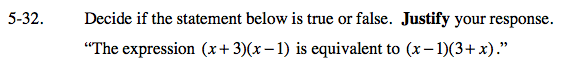5-32.

Missing problem for Book AC => Chapter Ch5 => Lesson 5.1.3 => Problem 5-32

Created from orphaned homework help problemThese expressions are equivalent because of the Commutative properties of Addition, x + 3 = 3 + x,
and because of the Commutative properties of Multiplication, (x + 3)(x − 1) = (x − 1)(x + 3).